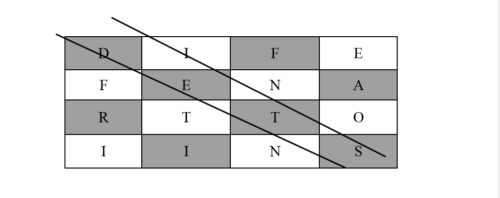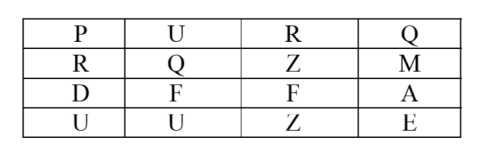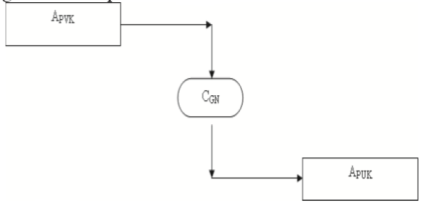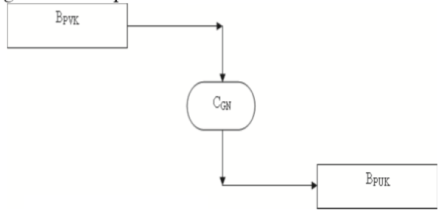Disclaimer: This is an example of a student written essay.

Any opinions, findings, conclusions or recommendations expressed in this material are those of the authors and do not necessarily reflect the views of UKEssays.com.

# Overview of Cryptographic Techniques

 ✅ Paper Type: Free Essay ✅ Subject: Computer Science ✅ Wordcount: 1600 words ✅ Published: 29th Mar 2018

Gunasekaran, Priya Dharshini

Cryptographic Techniques

The backbone of many organization is the electronic communications. Most of the information are communicated online. Hence the important informations are to be maintained confidentially. To maintain the highly confidential information, a secure and robust system is required. Som and Banerjee (2013, p. 1) states that “Cryptography is the science of making communication unintelligible to everyone except the intended receiver(s).” Message secrecy and reliability, sender verification are the main feature provided by cryptography. The major functionality of cryptography are encryption and decryption. Encryption is a process of changing the simple text into a cipher text. Decryption is the reverse process, changing the cipher text into a simple readable text. The encryption and the decryption are processed based on the key shared between the sender and the receiver. Sender uses the key to encrypt the information, whereas the receiver uses the key to decrypt the encrypted information. Kester and Danquah says that symmetric and asymmetric are the two main cryptographic algorithms. Symmetric key algorithm exchanges the public key which is used to encrypt and decrypt the message. Asymmetric algorithm provides more security than the symmetric algorithm since it uses both public and private keys for generating the cipher text. Various cryptographic techniques have been discovered based on the symmetric and asymmetric algorithms. This paper discuss the three major cryptographic techniques, square matrix algorithm, public key exchange algorithm using matrix, and asymmetric key algorithm using arithmetic equations.

If you need assistance with writing your essay, our professional essay writing service is here to help!

The most basic cryptographic technique is square matrix algorithm. Som and Banerjee says that in this technique the data is placed in the square matrix as in Figure 1 (2013, p. 1). The authors says that a matrix is considered based on the size of the simple text and the letters in the simple text are placed diagonally in the matrix (2013). Som and Banerjee explains that the key is generated by adding the position values of the letters in the diagonal position (p. 1).The author explains that the letters in the square are read serially and the position values are added with the key value obtained. Som and Banerjee says that for the each of the position value obtained the chipper text is generated (p. 2). Som and Banerjee explains this algorithm taking the simple text as “DIFFERENTIATIONS” (p. 2).Figure 1. Plain text are placed into a square matrix (Som, Banerjee, 2013, p. 2)

Som and Banerjee describes that the key is generated as addi ng the position value of ‘D’, position value of ‘E’, position value of ‘T’, position value of ‘S’ which results as 38, the value of the each letters are like A being1 up to Z being 26 (2013, p. 2). Som and Banerjee states that the intermediate chipper text generated by adding the key value with the each of the position value is “PURQRQZMDFFAUUZE” as in Figure 2 (p. 2).Figure 2. The matric table of the intermediate cipher text (Som, Banerjee, 2013, p. 2)

According to Som and Banerjee intermediate chipper text is converted into binary form and the sub sectioned (2013, p. 3). Som and Banerjee says that the binary converted data is combined with the next set of blocks (p. 3). This procedure is followed for all the sub sectioned blocks. In this way the each of the block is formed resulting in the actual cipher text. In this example the actual cipher text is 640 680 656 2592 2624 2592 180 152 24159191040 20401094936 70 260 680 680 720 517. Som and Banerjee explain that the decryption of the cipher is converting the number into its binary form (p. 3). The authors also explains that the binary data is then divided into a set of blocks and the individual letter for the each block is obtained (2013). Som and Banerjee states that the plain text is obtained by subtracting the position value of the intermediate text with the shared key value (2013). This method is one of the easiest method to secure the information.

Public key exchange algorithm using matrix is another cryptographic techniques. Kester explains the algorithm with help of two parties A and B. According to Kester an individual private key will be chosen for the parties A and B as APVK and BPVK respectively (2012). The author syas that the public key of the party A and B, APUK and BPUK is obtained from the private key using an algorithm, considering the algorithm being CGN as shown in the Figure 3 and Figure 4 respectively (2012).Figure 3. Public key generation for the party A (Kester, 2012, p. 79)Figure 4. Public key generation for the party B (Kester, 2012, p. 79)

Kester also says that the public key, APUK and BPUK, is shared between both the parties (2012, p. 79). According to author the private key is not exchanged between the parties (2012, p. 79). Kester describes that the plain text is encrypted by the party A and sent to party B (2012, p. 79). The author also says that the encryption is performed using the private key of party A and the public of the party B, since the public is shared with the party A (2012). Kester explains that the encrypted text is sent to the party B (2012, p. 79). Kester states that the message received by party B is decrypted using the private key of the party A (2012, p.79). The author describes that the public key is primarily used for authentication and private keys are used for encryption (2012). According to Kester, “The decryption of the message of a particular cipher text will only be possible if and only if the message sent is intended to be read by that recipient” (2012, p. 80). This algorithm is the basic functionality for all encryption and decryption methods.

Our academic experts are ready and waiting to assist with any writing project you may have. From simple essay plans, through to full dissertations, you can guarantee we have a service perfectly matched to your needs.

Asymmetric key algorithm using arithmetic equations is a mathematical cryptographic technique. According to Kester and Danquah (2012, p. 71), the intermediate cipher text is generated using twelve equation and the main cipher text is generated using the shared key. Kester and Danquah state that there are two keys for a complete cryptographic procedure which are public key for authentication, private key for encryption and decryption (2012). Kester and Danquah describes that a software was developed based on the public key and the private key (2012). Kester and Danquah also states that only eight string of data can be used by the software (2012). Therefore this algorithm is explains the method to generate the chipper text using the software.

According to Kester and Danquah, a constant value a chose between which below the number of letter of the plain text (2012).The authors state that the public key if the indivual part is generated by applying the mod function on the constant value (2012). The authors also say that the private key is any value chose below the number of letter in the plain text (2012). The authors state that the cipher text is generated using on the private and the public keys using the mod function (2012).

The authors state that the public key is shared between two users and the private key is generated for individual users (2012, p. 71). Kester and Danquah says that the decryption is the reverse process of the encryption following the algorithm form the step 12 to the step 1 (2012). The authors also say that the public generated id unique for sender and the receiver and is dependent on the private key of the sender and the receiver (2012, p. 72). Kester and Danquah state that “The cipher for the public key from the private key and vice versa depends on the function used to generate keys” (2012, p. 73). This algorithm is an arithmetic cryptographic techniques generating the cipher text based on the position of the text place.

Square matrix algorithm, public key exchange algorithm using matrix, and asymmetric key algorithm using arithmetic equations are the three major cryptographic techniques. They are several different cryptographic methods to secure the message delivered through the internet. Mukesh and Subashini says that biometric algorithm has been implemented widely and is proved that the data is secured effectively (2012, p. 19).

References

Kester, Q. A., (2012). A Public-Key Exchange Cryptographic Technique using Matrix, IEEE, 978-1-4673-4789-1/12, 78-81.

Kester, Q. A., & Danquah, P., (2012). A Novel Cryptographic key technique, IEEE, 978-1-4673-4789-1/12, 70-73.

Mukesh, R., & Subashini, V. J., (2012). Fingerprint based Authentication system using threshold visual cryptographic technique, IEEE, IBSN: 978-81-909042-2-3, 16-19.

Som, S., & Banerjee, M., (2013). Cryptographic Technique by Square Matrix and Single Point Crossover on Binary Field, IEEE, 978-1-4673-2821-0/12, 106.

View all

## DMCA / Removal Request

If you are the original writer of this essay and no longer wish to have your work published on UKEssays.com then please:

Related Services

Prices from

\$USD152

Approximate costs for:

• 1000 words
• 7 day deliveryHumanity University

Dedicated to your worth and value as a human being!

Related Lectures